Processing ......FreeComputerBooks.com Links to Free Computer, Mathematics, Technical Books all over the World

Data Science Algorithms in a Week: Top 7 Algorithms for Computing, Data Analysis, and Machine Learning

• Title: Data Science Algorithms in a Week: Top 7 Algorithms for Computing, Data Analysis, and Machine Learning
• Author(s) David Natingga
• Publisher: Packt Publishing (August 16, 2017)
• Paperback: 210 pages
• eBook: PDF
• Language: English
• ISBN-10/ASIN: 1787284581
• ISBN-13: 978-1787284586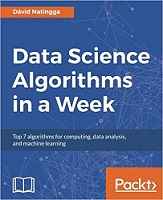Book Description

This book will address the problems related to accurate and efficient data classification and prediction. Over the course of 7 days, you will be introduced to seven algorithms, along with exercises that will help you learn different aspects of machine learning. You will see how to pre-cluster your data to optimize and classify it for large datasets. You will then find out how to predict data based on the existing trends in your datasets.

It covers algorithms such as: k-Nearest Neighbors, Naive Bayes, Decision Trees, Random Forest, k-Means, Regression, and Time-series. On completion of the book, you will understand which machine learning algorithm to pick for clustering, classification, or regression and which is best suited for your problem.

• Find out how to classify using Naive Bayes, Decision Trees, and Random Forest to achieve accuracy to solve complex problems
• Identify a data science problem correctly and devise an appropriate prediction solution using Regression and Time-series
• See how to cluster data using the k-Means algorithm
• Get to know how to implement the algorithms efficiently in the Python and R languages
• David Natingga is a mathematician and theoretical computer scientist of a Slovak descent working on the mathematics of a mind.
Reviews, Rating, and Recommendations: Related Book Categories: Read and Download Links:Similar Books:
•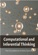Computational and Inferential: The Foundations of Data Science

Step by step, you'll learn how to leverage algorithmic thinking and the power of code, gain intuition about the power and limitations of current machine learning methods, and effectively apply them to real business problems.

•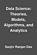Data Science: Theories, Models, Algorithms, and Analytics

It provides a bucket full of information regarding Data Science, covers a wide variety of sections by giving access to theories, data science algorithms, tools and analytics. You'll explore the right approach to best practices to guide you along the way.

•Python Data Science Handbook: Essential Tools (Jake VanderPlas)

Several resources exist for individual pieces of this data science stack, but only with the Python Data Science Handbook do you get them all - IPython, NumPy, Pandas, Matplotlib, Scikit-Learn, and other related tools.

•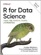R for Data Science: Visualize, Model, Transform, Tidy, Import

This book teaches you how to do data science with R: You'll learn how to get your data into R, get it into the most useful structure, transform it, visualize it and model it, how data science can help you work with the uncertainty and capture the opportunities.

•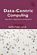A Data-Centric Introduction to Computing (Kathi Fisler, et al)

This book is an introduction to computer science. It will teach you to program, and do so in ways that are of practical value and importance. It uses a data-centric approach: data centric = data science + data structures.

•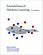Foundations of Machine Learning (Mehryar Mohri, et al)

This book is a general introduction to machine learning. It covers fundamental modern topics in machine learning while providing the theoretical basis and conceptual tools needed for the discussion and justification of algorithms.

Book Categories
 :All CategoriesRecent BooksMiscellaneous BooksComputer LanguagesComputer ScienceData Science/DatabasesElectrical EngineeringJava and Java EE (J2EE)Linux and UnixMathematicsMicrosoft and .NETMobile ComputingNetworking and CommunicationsSoftware EngineeringSpecial TopicsWeb Programming
Other Categories